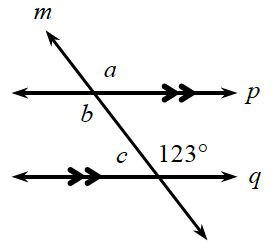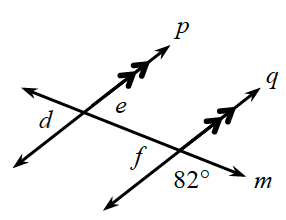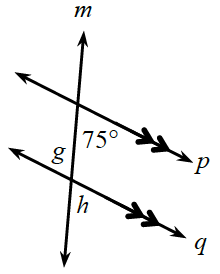### Home > INT1 > Chapter 1 > Lesson 1.2.1 > Problem1-42

1-42.

Recall what you learned about angle relationships in previous courses. (See the Math Notes box in this lesson if you need a reminder.) Determine the measures of the labeled angles. The double arrowheads indicate that the pair of lines is parallel.

All three of these can be solved using multiple techniques related to parallel lines.

1.$\angle a$: Corresponding angle with $123º$, so $m\angle a = 123º$ .
$\angle b$: $\angle a$ and $\angle b$ are vertical angles, so they have equal measures. Therefore, $m\angle b = 123º$.
$\angle c$: $\angle c$ and $123º$ form a pair of supplementary angles, so $m\angle c = 57º$.

1.$\angle f$ and $82º$ form a pair of supplementary angles. How are $\angle e$ and $\angle f$ related?

1.Since there are parallel lines, look for relationships between pairs of angles such as alternate interior, corresponding, or same-side interior.